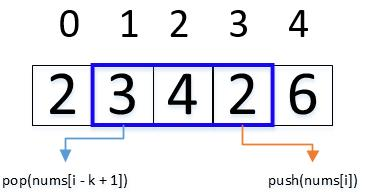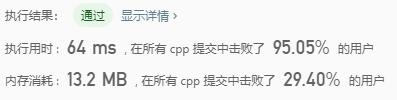# Leetcode 239：滑动窗口最大值

## 示例

————— —–
[1 3 -1] -3 5 3 6 7 3
1 [3 -1 -3] 5 3 6 7 3
1 3 [-1 -3 5] 3 6 7 5
1 3 -1 [-3 5 3] 6 7 5
1 3 -1 -3 [5 3 6] 7 6
1 3 -1 -3 5 [3 6 7] 7

## 解题思路

void push(int n);//在队尾插入一个元素void pop(int n);//队头元素如果是n删除它int max();//返回当前队列中的最大值

 vector<int> maxSlidingWindow(vector<int>& nums, int k) { vector<int> result; if (nums.empty()) return result; Solution win; for (int i = 0; i < nums.size(); i++) { if (i < k - 1) win.push(nums[i]);//先填满窗口前k-1个元素 else { //插入队尾元素，else后面这三句话相当于窗口滑动且找到最大值push给result win.push(nums[i]); result.push_back(win.max()); win.pop(nums[i - k + 1]); } } return result; }void push(int n) { while (!data.empty() && data.back() < n) data.pop_back(); data.push_back(n); }int max() { return data.front();}

## 代码实现

class Solution {public: private: deque<int> deq;public: void push(int k) { while (!deq.empty() && deq.back() < k) deq.pop_back(); deq.push_back(k); } int max() { return deq.front(); } void pop(int k) { if (!deq.empty() && deq.front() == k) deq.pop_front(); } vector<int> maxSlidingWindow(vector<int>& nums, int k) { vector<int> result; if (nums.empty()) return result; Solution win; for (int i = 0; i < nums.size(); i++) { if (i < k - 1) win.push(nums[i]);//先填满窗口前k-1个元素 else { win.push(nums[i]); result.push_back(win.max()); win.pop(nums[i - k + 1]); } } return result; }};

## 复杂度